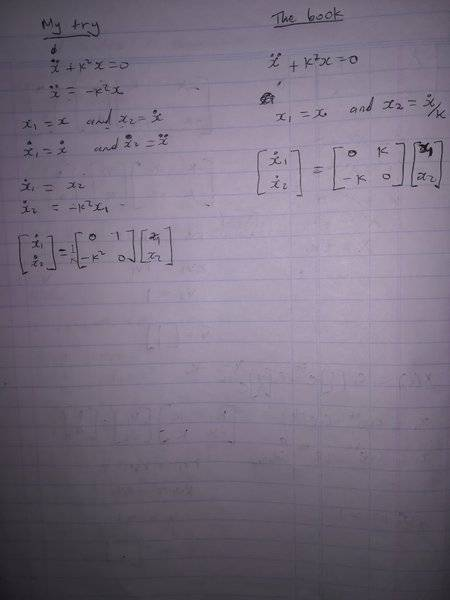# Second order ordinary differential equation to a system of first order

• I
I tried to convert the second order ordinary differential equation to a system of first order differential equations and to write it in a matrix form. I took it from the book by LM Hocking on (Optimal control). What did I do wrong in this attachment because minediffers from the book?. I've attached both the book solution and mine. Thanks.

#### Attachments

tnich
Homework Helper
I tried to convert the second order ordinary differential equation to a system of first order differential equations and to write it in a matrix form. I took it from the book by LM Hocking on (Optimal control). What did I do wrong in this attachment because mineView attachment 226158 differs from the book?. I've attached both the book solution and mine. Thanks.
I don't think there is anything wrong with your way (except for the ##\frac 1 k## you have penciled in front of the matrix in your equation). It still leads to the same solution to the differential equation. Your way does require fiddling with the constants a little more to get to that solution, which may be why your textbook gives the particular form you found there.

BvU